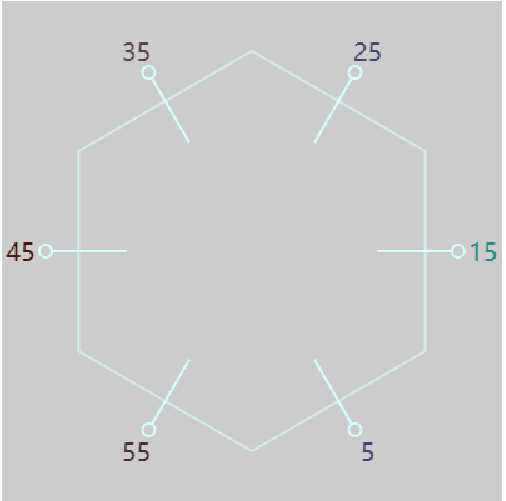Canvas -- 角度与弧度

角度

其他表示方法：

1. 90° 即 π / 2。
2. 60° 即 π / 3.
3. 180° 即 π。

Canvas 中的弧度。

同角度一样。计算公式：假设当前弧度为 deg，( js 中的 π 用 Math.PI 表示)

** 弧度 = deg * Math.PI / 180 **

以下canvas实例：通过角度去计算圆中某一点:

• 直接求得 x轴长度 , y轴长度需要使用 Math实例方法；Math.sin(),Math.cos();

let x = Math.cos( deg * Math.PI / 180) * r; // 已知半径和角度，求 x 轴的长度
let y = Math.sin( deg * Math.PI / 180) * r; // 已知半径和角度，求 y 轴的长度Canvas -- 角度与弧度

(0)
(0)© 2014 mamicode.com 版权所有 京ICP备13008772号-2